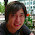## Thursday, November 1, 2012

### Building a Gesture Recognizer - Part 1 Dynamic Time Warping

I recently noticed a lot of people want to do "something with gestures" but don't know how to start.
So I thought writing a two part tutorial on how to recognize gestures. The first part will
describe the problems of gesture recognition and will describe a very simple algorithm, while
the second part will give the insights in what most gesture recognition systems currently use.

The goal in this tutorial is to understand a common problem in gesture recognition called warping
and to build a simple, distance based gesture recognizer.

So you want to recognize gestures. For simplicity let us assume you want to recognize gestures
performed with a mouse. If you record the position of your mouse over time, you will
have a two dimensional time series. In the picture below you can see an example.
I recorded a gesture and plotted the x and y position separately.TOP: a recorded gesture, CENTER: the position in x - axis,  BOTTOM: the position in y - axis.

If we want to build a recognizer we have to collect data first. For example if we have four different
gestures we could record ten users performing each gesture twenty times. So the resulting data set includes 800 recordings. Now one problem is that the examples for a gesture will not
exactly look the same. For example some users will perform the gesture faster and slower resulting
in an effect called "warping". One solution to this problem is to align the time series. An alignment
is the association of each point in one time series to another point in the other time series.Two examples of the same gesture (only x - axis). One example is warped. The purple lines show the alignment of the two time series.

In order to compare two warped time series we will use Dynamic Time Warping.
Dynamic Time Warping compares two time series while accounting for the warp using Dynamic Programming. In general the warping is resolved by accounting for added samples (the second example is performed slower) or deleting samples (the second example is performed faster.

The distance is calculated by constructing an M x N matrix V, where M is the length of one time series X = x1... xM and N the length of another time series Y = y1 ... yN. In our case xi and yj are two dimensional vectors (the position of the mouse). In each matrix position v(i,j)
we minimize the distance comparing the time series up to position i in X and position j in Y by the following recursion:

while v(0,0) is initialized as 0 and v(i,0) as well as v(0, j) are initialized as infinity.
Now that we have a distance measure we can implement a very simple recognizer.
We use the Nearest Neighbor Classifier using the distance described above.
For a gesture we have recorded we will calculate the Dynamic Time Warping distance
to each example in our pre recorded gesture set. The gesture associated with the example
that has the minimum distance is the recognized gesture.Two gestures shown in the distance space from a new example.
One important question to ask is "What happens when the new example is not a gesture".
In that case we apply a threshold on the gesture. If the distance is too high (above the threshold)
we will not consider it as anything.

So we built a very simple recognizer. The implementation should be straight forward. In on of the next posts I will walk through gesture recognition using Hidden Markov Models. Since Hidden Markov Models are not easy to implement we will use an existing Toolkit called HTK3. I will write a step by step tutorial.

1.Hi I am doing a project on indian sign language recognition. (need to recognize the word from the sign performed)
I have segmented the two hand n head region.
Is it possible to use DTW algorithm to find the word from the traces that we get by doing an action.

1.Hello Anton,
I am not sure what you mean with "n head region" and " find the word from the traces that we get by doing an action".
You can write me an email if you like to discuss this further.

2.Thanks for the article!